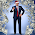## Monday, June 22, 2015

### Quantized Spacetime: MpRp=2h/(pi*c)=MeRe

$$m_pr_p={2h\over\pi c}=m_er_e$$

The product of matter and space (distance) are both quantized.
Spacetime is quantized.

This is quantized torque-spin-coriolis-spacetime-momentum!

For the higher energy levels, n, I will be adding the appropriate extension, then from there, multi-nucleon (proton, neutron) nucleus, and more.... it's a whole series of fields that come from this founding equation, which is clearly apparent that it is simply a matter of going through the math and analysis to complete each and every field. (physics, cosmology, biology, chemistry, science, life, memory, consciousness... and so on..., like:
$$m_pr_p={2nh\over\pi c}=m_er_e$$
or some function of n, f(n), that is geometric and models the electron states:
$$m_pr_p={2f(n)h\over\pi c}=m_er_e$$
I'll check on how well this models the different energy states next as I dig into completing and checking this formulation.

Founding Field: Quantum Gravity.
Mark Eric Rohrbaugh and Lyz Starwalker
©2015 Mark Eric Rohrbaugh ©2015 Lyz Starwalker

1.This is the unification of physics. -mr -ls

2.this correct for the mistakes of Bohr, et al, and connects Einstein's work to Planck's work.

3.for the higher energy levels, n, I will be adding the appropriate extension, then from there, multi nucleon (proton, neutron) nucleus, and more.... it's a whole series of fields that come from this founding equation, which is clearly apparent that it is simply a matter of going through the math and analysis to complete each and every field. (physics, cosmology, biology, chemistry, science, life, memory, consciousness... and so on...

4.Founding Field: Quantum Gravity. Mark Eric Rohrbaugh and Lyz Starwalker

5.Founding Field: Quantum Gravity. Mark Eric Rohrbaugh and Lyz Starwalker

6.This stuff relates directly to Dan Winter's work.

7.this is standard quantum work simply reworked with the correct founding assumptions, like Nassim Haramein has been saying.

8.Brilliant and ground breaking Mark & Lyz.

1.Thanks Grayham. It took Lyz Starwalker's work, Haramein's, Rauscher's, and much more to tie together the electron and proton to complete the Standard Model (SM is now the CSM). The covenant is the electron arc. Shoosh, don't tell anyone. I don't know how Lyz came up with it, however, I struggled since 1987 or so attempting to find a reason to equate MpRp with MeRe and setting both equal to 4LM (4=Favre, L=Planck Length, M=Planck Mass). I hope Nassim Haramein and Marko Rodin et al find this useful. I am confident Elizabeth Rauscher sees the value in this work of Lyz Starwalker. More later as this is ongoing, and we still have to face #CERN , LOL !!!

Watch the water. 🦆